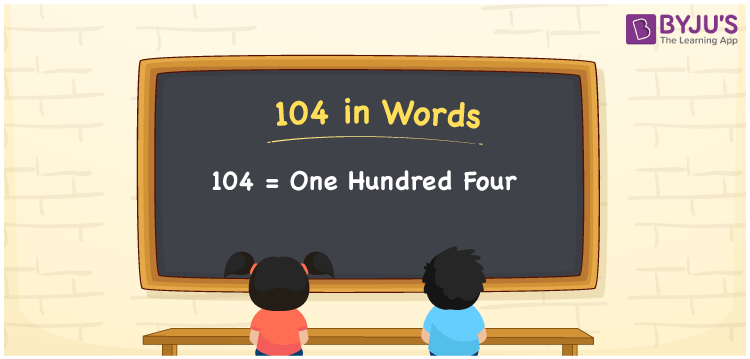# 104 in Words

In English words, 104 is written as One Hundred Four. For example, if you purchased a cap worth Rs 104, then you can say, “I purchased a cap worth Rupees One Hundred Four”. Any number can be converted into words easily with the help of a place value of the given number. Hence, we should make use of a place value chart to write the number names. 104 is a cardinal number since it represents a specific quantity or value. In this article, let us learn how to spell the number 104 in English words.

 104 in Words One Hundred Four One Hundred Four in numerical form 104

## 104 in English Words

Students must learn the numbers in words using the English alphabet since English is the widely used language in the education field. Therefore, the number 104 in English is read as One Hundred Four.## How to Write 104 in Words?

We use a place value chart to convert the number 104 in word form. Let us create a table of 3 columns to represent the place value chart for the number 104 as it is a three-digit number.

 Hundreds Tens Ones 1 0 4

Hence, we can write the expanded form as:

1 x Hundred + 0 x Ten + 4 x One

= 1 x 100 + 0 x 10 + 4 x 1

= 100 + 0 + 4

= 100 + 4

= 104

= One Hundred Four

Therefore, 104 in words is written as One Hundred Four

Interesting way of writing 104 in words

1 = One

10 = Ten

104 = One Hundred Four

Thus, the word form of the number 104 is One Hundred Four

104 is a natural number that precedes 105 and succeeds 103

• 104 in words – One Hundred Four
• Is 104 an odd number? – No
• Is 104 an even number? – Yes
• Is 104 a perfect square number? – No
• Is 104 a perfect cube number? – No
• Is 104 a prime number? – No
• Is 104 a composite number? – Yes

## Frequently Asked Questions on 104 in Words

Q1

### How to write 104 in words?

104 in words is written as One Hundred Four.
Q2

### Simplify 89 + 15, and express in words.

Simplifying 89 + 15, we get 104. Hence, 104 in words is One Hundred Four.
Q3

### Write One Hundred Four in numbers.

One Hundred Four in numbers is 104.